# TTL Logic Gates

## Digital Circuits

• #### Question 1

Counting practice: count from zero to thirty-one in binary, octal, and hexadecimal:• #### Question 2

 Don’t just sit there! Build something!!

Learning to analyze digital circuits requires much study and practice. Typically, students practice by working through lots of sample problems and checking their answers against those provided by the textbook or the instructor. While this is good, there is a much better way.

You will learn much more by actually building and analyzing real circuits, letting your test equipment provide the “answers” instead of a book or another person. For successful circuit-building exercises, follow these steps:

1. Draw the schematic diagram for the digital circuit to be analyzed.
2. Carefully build this circuit on a breadboard or other convenient medium.
3. Check the accuracy of the circuit’s construction, following each wire to each connection point, and verifying these elements one-by-one on the diagram.
4. Analyze the circuit, determining all output logic states for given input conditions.
5. Carefully measure those logic states, to verify the accuracy of your analysis.
6. If there are any errors, carefully check your circuit’s construction against the diagram, then carefully re-analyze the circuit and re-measure.

Always be sure that the power supply voltage levels are within specification for the logic circuits you plan to use. If TTL, the power supply must be a 5-volt regulated supply, adjusted to a value as close to 5.0 volts DC as possible.

One way you can save time and reduce the possibility of error is to begin with a very simple circuit and incrementally add components to increase its complexity after each analysis, rather than building a whole new circuit for each practice problem. Another time-saving technique is to re-use the same components in a variety of different circuit configurations. This way, you won’t have to measure any component’s value more than once.

• #### Question 3

Identify each of these logic gates by name, and complete their respective truth tables:• #### Question 4

The simplest type of digital logic circuit is an inverter, also called an inverting buffer, or NOT gate. Here is a schematic diagram for an inverter gate constructed from bipolar transistors (transistor-to-transistor-logic, also known as TTL), shown connected to a SPDT switch and an LED:The left-most transistor in this schematic is actually not being used as a transistor, but rather it functions as a “steering diode” network, like this: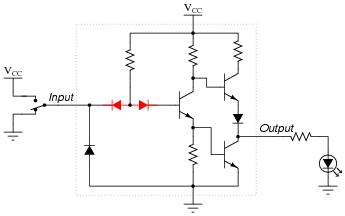Determine the status of the LED in each of the input switch’s two positions. Denote the logic level of switch and LED in the form of a truth table: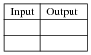• #### Question 5

The following is an internal schematic of a TTL logic gate. Based on your analysis of the transistor circuit, determine what type of gate (AND, OR, NAND, NOR, XOR, etc.) it is:Hint: the double-emitter transistor is being used as a pair of diodes, and not as an amplifying device!• #### Question 6

A very important concept to understand in digital circuitry is the difference between current sourcing and current sinking. For instance, examine this TTL inverter gate circuit, connected to a load:The output circuitry of this particular gate is commonly referred to as “totem-pole,” because the two output transistors are stacked one above the other like figures on a totem pole. Is a gate circuit with a totem-pole output stage able to source load current, sink load current, or do both?

• #### Question 7

A very important concept to understand in digital circuitry is the difference between current sourcing and current sinking. For instance, examine this open-collector TTL inverter gate circuit, connected to a load:Open-collector gates are specially designated in their schematic symbols by a marker within the gate shape: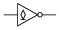Is this gate circuit able to source load current, sink load current, or do both?

• #### Question 8

Based on an analysis of a typical TTL logic gate circuit (consult a datasheet for a TTL logic gate if you need an internal schematic diagram for a gate circuit), determine what logic state is “assumed” by a TTL gate input when left “floating” (disconnected).

What ramification does this have for us when choosing input devices for TTL logic gates? If, for instance, we wished to use a single-pole, single-throw (SPST) switch as the input device for a TTL logic gate, what is the best way to connect such a device to a TTL input? Should the switch connect the TTL input to VCC when closed, or should it connect the input to VEE when closed? Why does it matter? Explain your answer in detail.

• #### Question 9

A logic probe is a very useful tool for working with digital logic circuits. It indicates “high” and “low” logic states by means of LED’s, giving visual indication only if the voltage levels are appropriate for each state.

Here is a schematic diagram for a logic probe built using comparators. Each comparator has a threshold adjustment potentiometer, so that it may be set to indicate its respective logic state only if the signal voltage is well within the range stated by the logic manufacturer: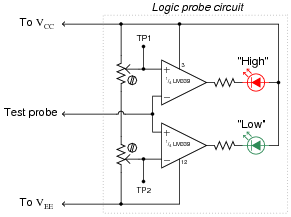When this logic probe circuit is connected to the VCC and VEE power supply terminals of a powered TTL circuit, what voltage levels should test points TP1 and TP2 be adjusted to, in order for the probe to properly indicate “high” and “low” TTL logic states? Consult a datasheet for the quad NAND gate numbered either 74LS00 or 54LS00. Both are legacy TTL integrated circuits.

• #### Question 10

A student builds the following digital circuit on a solderless breadboard (a “proto-board”):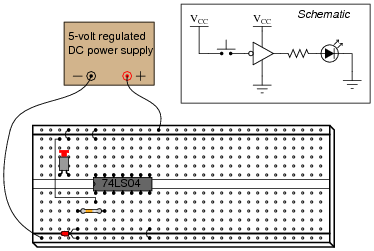The DIP circuit is a TTL hex inverter (it contains six “inverter” or “NOT” logic gates), but only one of these gates is being used in this circuit. The student’s intent was to build a logic circuit that energized the LED when the pushbutton switch was unactuated, and de-energized the LED when the switch was pressed: so that the LED indicated the reverse state of the switch itself. However, in reality the LED fails to energize no matter what state the switch is in.

First question: how would you use a multimeter as a logic probe to check the logic states of points in this circuit, in order to troubleshoot it?

Second question: suppose you checked the logic states of pin #1 on the IC, for both states of the switch (pressed and unpressed), and found that pin #1 was always “high”. How does this measurement indicate the student’s design flaw in this circuit? How would you recommend this design flaw be corrected?

• #### Question 11

Draw the paths of all currents in this circuit with the input in a “low” state:Now, draw the paths of all currents in this circuit with the input in a “high” state:Where is the power supplied for each LED? What relationship is there between the load current (LED) and the gate input current (through the SPDT switch)?

Also, explain how you would calculate the values for appropriate LED current-limiting resistors in this circuit.

• #### Question 12

The digital circuit shown here is a unanimous-yea vote detector. Votes are cast by eight different voters by the setting of switches in either the closed (yea) or open (nay) positions. According to the logic function provided by the TTL gates, the LED will energize if and only if all switches are closed:As is common in digital circuit schematics, the power supply (VCC) is omitted for the sake of simplicity. This is analogous to the omission of power supply connections in many operational amplifier circuit schematics.

If we were to draw a truth table for this circuit, how large (number of rows and columns) would the table have to be?

Suppose we wished to modify this circuit, such that an electromechanical bell would ring whenever a unanimous-yea vote was cast, rather than merely lighting a small LED. The bell we have in mind to use is rather large, its solenoid coil drawing 3 amps of current at a voltage of 12 volts DC: well beyond the final gate’s ability to source. How could we modify this circuit so that the final gate is able to energize this bell instead of just an LED?

• #### Question 13

Totem-pole TTL gates usually differ greatly in their maximum source current versus maximum sink current (IOH versus IOL). Identify which current rating is usually greater, and also explain why this is.

• #### Question 14

In high-speed digital circuits, a very important logic gate parameter is propagation delay: the delay time between a change-of-state on a gate’s input and the corresponding change-of-state on that gate’s output. Consult a manufacturer’s datasheet for any TTL logic gate and report the typical propagation delay times published there.

Also, explain what causes propagation delay in logic gates. Why isn’t the change in output state instantaneous when an input changes states?

• #### Question 15

Logic gates are limited in the number of gate inputs which one output can reliably drive. This limit is referred to as fan-out:Explain why this limit exists. What is it about the construction of TTL logic gates that inherently limits the number of TTL inputs that any one TTL output can drive? What might happen if this limit is exceeded?

Locate a datasheet for a TTL gate and research its fan-out limit. Note: this number will vary with the particular type of TTL referenced (L, LS, H, AS, ALS, etc.).

• #### Question 16

Explain why it is generally a very bad design practice to connect the outputs of different logic gates together, like this:However, there are certain specific circumstances in which “paralleling” gate outputs is acceptable. For instance, it is okay to parallel two or more inverters, like this:No damage will be done if open-collector gate outputs are paralleled, either (although the resulting logic function may be strange):And finally, gates that have tri-state outputs may also have their outputs paralleled if certain precautions are taken:What, specifically, causes gates to be damaged by “paralleling” their outputs? Generally speaking, what principle must be followed in order to “parallel” logic gate outputs without risk of damage? Explain how each of the three acceptable “paralleled” scenarios shown here meet this criterion.

Suggestion: the issue of multiple gates having to output logic voltage signals onto common conductors (“busses”) is called bus contention. Try looking for this term in your research to see what useful information you find on paralleled gates!

• #### Question 17

An important parameter of logic gate circuitry is noise margin. What exactly is “noise margin,” and how is it defined for logic gates?

Specifically, how much noise margin do digital circuits exclusively composed of TTL gates have?

Note: you will need to consult TTL gate datasheets to answer this question properly.

• #### Question 18

Predict how the operation of this logic gate circuit will be affected as a result of the following faults. Consider each fault independently (i.e. one at a time, no multiple faults):Diode D1 fails open:
Diode D1 fails shorted:
Diode D2 fails open:
Resistor R1 fails open:
Resistor R2 fails open:
Resistor R4 fails open:

For each of these conditions, explain why the resulting effects will occur.

• #### Question 19

Predict how the operation of this logic gate circuit will be affected as a result of the following faults. Consider each fault independently (i.e. one at a time, no multiple faults):Diode D1 fails open:
Diode D1 fails shorted:
Diode D2 fails open:
Resistor R1 fails open:
Resistor R2 fails open:
Transistor Q2 emitter terminal fails open:
Transistor Q3 emitter terminal fails open:

For each of these conditions, explain why the resulting effects will occur.

• #### Question 20

In TTL circuitry, one side of the DC power supply is usually labeled as “VCC”, while the other side is labeled as “VEE”. Why is this? What do the subscripts “CC” and “EE” represent?

• #### Question 21

True story: once upon a time, there was a machine shop containing a number of computer-controlled machine tools (lathes, mills, grinders, etc.), where one of the machines proved to be very “finicky” when starting. Sometimes, it would function properly when you pushed the “Start” button, and other times it refused to work at all. The problem was so bad, it got to the point where the machinists responsible for operating this tool became almost superstitious about it, performing a ritual dance before pressing the “Start” button, whimsically hoping to improve their luck.

An electrician was called to service this machine, but he could find nothing wrong with the electrical power circuitry. All of the high-voltage equipment (transformers, relays, motors, motor control circuits, etc.) seemed to be in good working order. The problem, whatever it was, resided within the machine’s electronic control computer. The computer was not sending the “start” signal to the motor control circuits when the “Start” button was pushed.

An electronics technician was called to troubleshoot the computer, and he was able to fix it in a matter of minutes. The problem, he said, was the computer’s DC power supply: the voltage regulator was out of adjustment. With just a twist of a potentiometer, the technician was able to “trim” the regulated voltage to 5.00 volts, right where it should be for TTL circuitry.

The power supply voltage was not very far from 5.00 volts before the technician adjusted it. How far is the supply voltage allowed to deviate for TTL logic circuits, and still have guaranteed proper operation? Consult one or more IC datasheets for legacy TTL logic circuits (not the newer high-speed CMOS 54HCxx and 74HCxx chips) to obtain your answer.

• #### Question 22

In this circuit, an AND gate is used to give a toggle switch control over the blinking of an LED: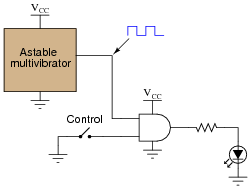The “astable multivibrator” is nothing more than an oscillator that produces a square-wave signal at a low frequency, at standard TTL voltage levels (0 and 5 volts).

Plot the output waveform for the gate (i.e. the voltage signal to the LED), given the following input conditions: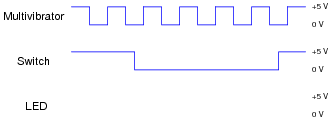Hint: it helps in your analysis of digital waveforms if you first write a truth table for the gate under consideration, for your reference.

• #### Question 23

What does it mean if you see a logic gate symbol in a schematic diagram with a strange-looking “S” figure drawn inside of it?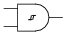• #### Question 24

For a true TTL gate (not high-speed CMOS), what is the default logic state of an input line that is left floating (neither connected to VCC nor Ground)? Explain why this is.